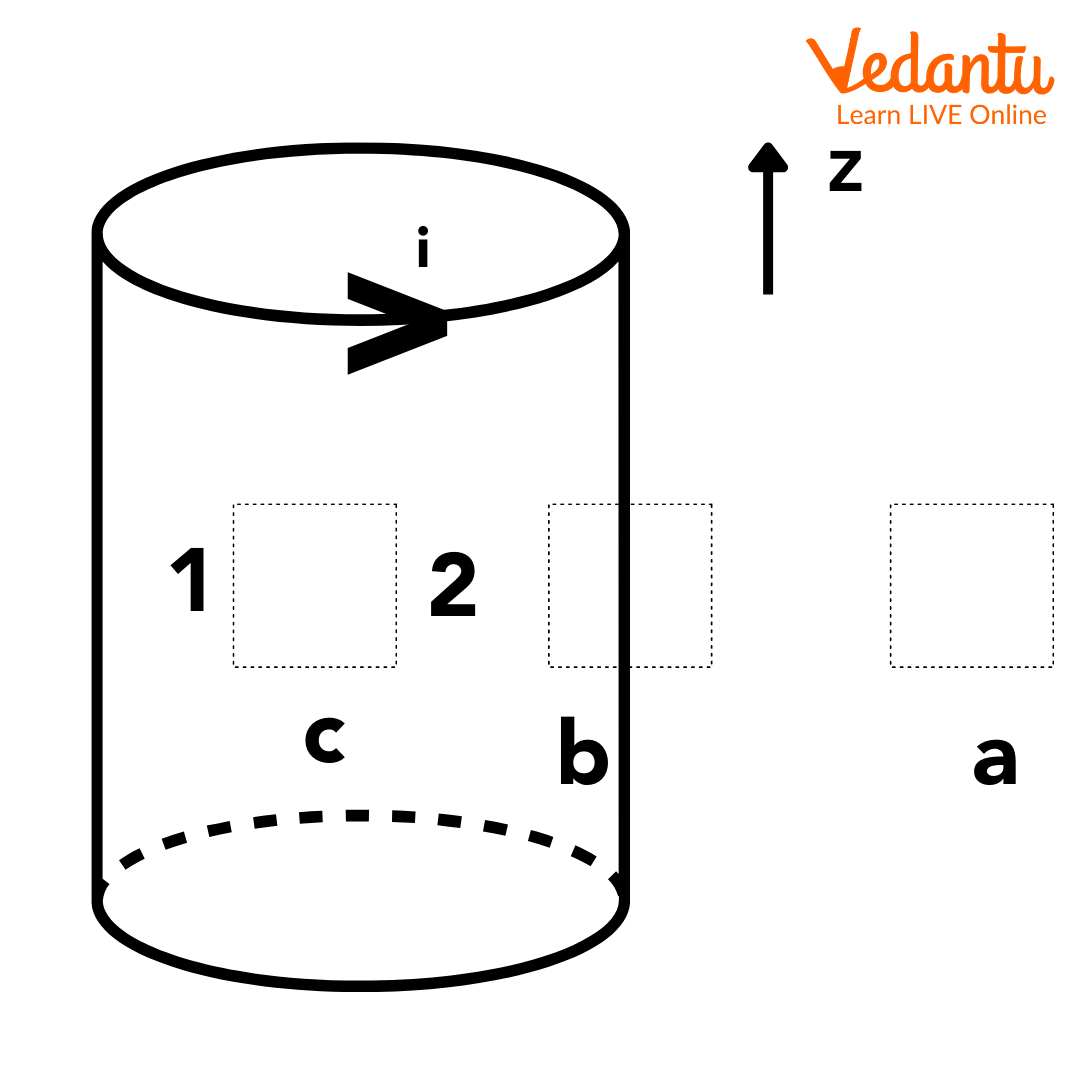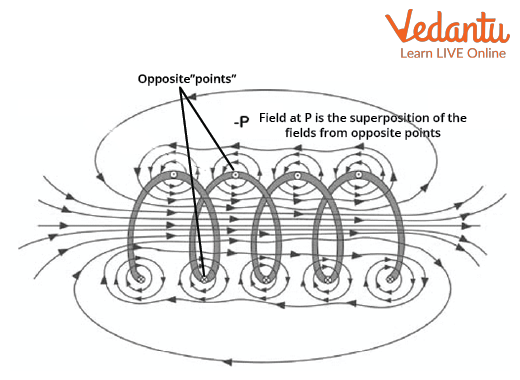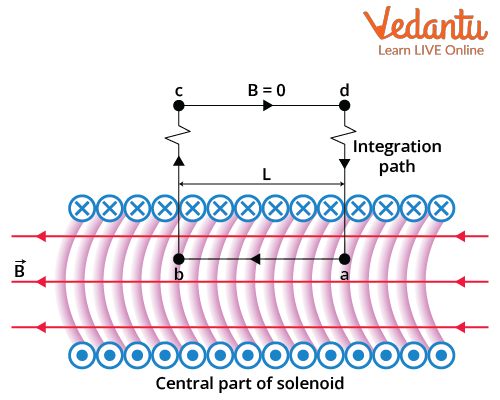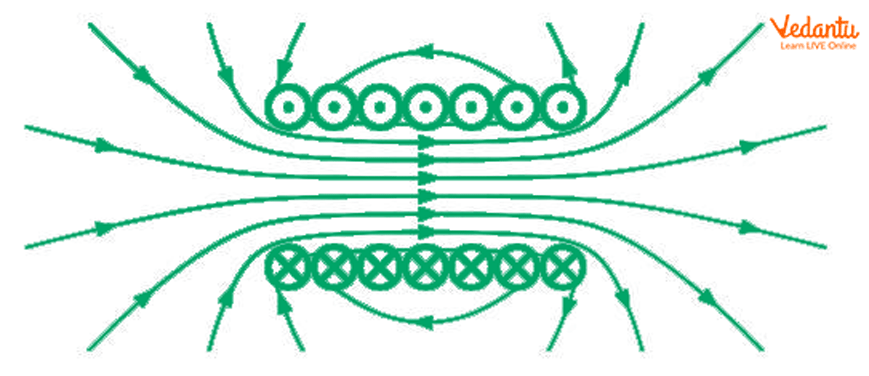Courses
Courses for Kids
Free study material
Free LIVE classes
More

# Solenoid Magnetic Field - Inside and Outside of Solenoid for JEELIVE
Join Vedantu’s FREE Mastercalss

## What is Solenoid?

A solenoid can be defined as a helical coil that is obtained by tightly bounding the copper wire to the cylindrical portion of the insulating material. When electric current flows through the solenoid, it creates a magnetic field.

Here, each turn of the solenoid is a circular loop when the turns are closely spaced, and the net magnetic field is the vector sum of the fields resulting from all the turns. The interior field becomes more uniform as the length of the solenoid increases. When the turns are closely spaced, and if the length is much greater than the radius of the turns, then it can be approximated as the ideal solenoid. The interior field is uniform over a great volume whereas the external field is zero.  An expression for the magnetic field in an ideal solenoid is obtained by Ampere’s Law.

## Magnetic Field Lines of Solenoid

The lines of force inside a solenoid are parallel to the axis of a solenoid and the magnetic field is uniform within the solenoid.Image: Magnetic Field Lines of Solenoid

### Magnetic Field Inside a Solenoid

This is a calculation of the magnetic field around a solenoid that is long enough to ignore fringe effects. The field at P points in the positive z-direction inside the solenoid (denoted by the dot) and in the negative z-direction outside the solenoid, (denoted by the cross) as shown in the diagram.Image: Magnetic Field inside the Solenoid

A solenoid equipped with three Ampèrian loops. This is demonstrated by using the right-hand thumb rule for the field around a wire. The curl of the fingers shows how the field behaves if we wrap our right hand around a wire with the thumb pointing in the direction of the current.

Because we are dealing with a long solenoid, all magnetic field components that are not pointing upwards cancel out due to symmetry. A similar cancellation occurs outside, and the field only points downwards.

Consider the imaginary loop c located inside the solenoid as shown in the image 1 above. We know from Ampère's law that the line integral of B (the magnetic field vector) around this loop is zero because it contains no electrical currents (also it can be assumed that the circuital electric field passing through the loop is constant under such conditions: a constant or constantly changing current through the solenoid). Because the field is pointing upwards inside the solenoid, the horizontal portions of loop c contribute nothing to the equation.

As a result, the integral of the side bc equals the integral of the side ad.Image: Magnetic Field of the Solenoid

Because the dimensions of the loop can be changed arbitrarily and still produce the same result, the only physical explanation is that the integrands are actually equal, implying that inside the solenoid, the magnetic field is radially uniform. But keep in mind that nothing prevents it from varying longitudinally, which it does.

## Magnetic Field Outside a Solenoid

A solenoid's magnetic field (cross-sectional view) is described using field lines. A similar argument can be used to conclude that the field outside the loop is zero. This result, which is true only near the centre of the solenoid where the field lines are parallel to its length, is significant because it shows that the field outside the solenoid is practically zero because the radii of the field outside the solenoid tend to infinity.

An argument can also be used to demonstrate that the field outside of the solenoid is zero. Magnetic field lines can only exist as loops; unlike electric field lines, they cannot diverge from or converge to a point because the magnetic field lines follow the longitudinal path of the solenoid inside, they must travel in the opposite direction outside of the solenoid in order to form a loop.Image: Magnetic Field Lines outside of the Solenoid

The volume outside the solenoid, on the other hand, is much larger than the volume inside, so the density of magnetic field lines outside is greatly reduced. Remember that the field outside is always the same. To conserve the total number of field lines, the field outside must decrease to zero as the solenoid lengthens.

## Solenoid Magnetic Field Formula

Now, we can consider the imaginary loop b. Calculate the line integral of B around the loop, where l is the length of the loop. Because the horizontal components vanish and the field outside is nearly zero, Ampère's Law yields:

$B l=\mu_{0} N I$

where $\mu_{0}$ represents the magnetic constant, N represents the number of turns, and I represents the current. This is the equation for a solenoid with no core. A ferromagnetic core, such as iron,  can increase the magnitude of the magnetic field in the solenoid. This expression formula is

$B=\mu_{0} \mu_{r} \dfrac{N}{l} I$

where $\mu_{r}$ is the relative permeability of the material used to make the core.  $\mu_{0} \mu_{r}$ is the core material's permeability ($\mu$) such that

$B=\mu_{0} nI$

where n represents the number of turns per unit length.

## Summary

A solenoid is a long wire wounded in the form of a helix that produces a uniform magnetic field. The magnetic field is uniform inside the solenoid and closes to zero outside the solenoid. We have used Ampere’s law to obtain an expression for the interior magnetic field in an ideal solenoid which states that the line integral of the magnetic field around a closed loop is proportional to the amount of current that is enclosed by the loop.

The magnitude of the magnetic field inside an ideal solenoid calculated from Ampere’s law is given as: Where n is the number of turns per unit length, I represent the current passing through it and is the constant of proportionality called the permeability of free space and has a value of T-m/A. The magnitude of the magnetic field will be constant inside a long solenoid.

Last updated date: 24th Sep 2023
Total views: 130.8k
Views today: 3.30k

## FAQs on Solenoid Magnetic Field - Inside and Outside of Solenoid for JEE

1. What is the magnitude of the magnetic field inside a long solenoid if 5A of current is passing through it? It is given that the length of the solenoid is  0.1m and it has 100 turns.

The number of turns per unit volume is obtained as:

$n=\dfrac{100}{0.1}=1000$ turns per metre

Hence, we can use the long solenoid formula.

\begin{align} &B=\mu_{0} n I \\ &B=4 \pi \times 10^{-7} \times 1000 \times 5 T \\ &B=6.28 \times 10^{-3} T \end{align}

2. What are the factors affecting solenoids?

Some of the important factors which affect the solenoid are given below:

(i) Number of turns per unit length in the solenoid:

The magnetic field produced in a solenoid is directly proportional to the number of turns, i.e., for a large number of turns, the magnetic field produced will be large.

(ii) Strength of the current in the coil of the solenoid:

The strength of the current in the coil decides the strength of the magnetic field produced; the larger the current is, the greater the magnetic field produced.

(iii) Material inside the solenoid:

For better performance of solenoid, highly permeable material is used as core.